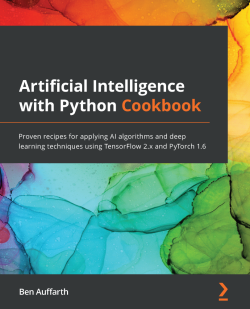### Artificial Intelligence with Python Cookbook

By Ben Auffarth
• Constantly updated with 100+ new titles each month
• Breadth and depth in over 1,000+ technologies

Artificial intelligence (AI) plays an integral role in automating problem-solving. This involves predicting and classifying data and training agents to execute tasks successfully. This book will teach you how to solve complex problems with the help of independent and insightful recipes ranging from the essentials to advanced methods that have just come out of research.

Artificial Intelligence with Python Cookbook starts by showing you how to set up your Python environment and taking you through the fundamentals of data exploration. Moving ahead, you’ll be able to implement heuristic search techniques and genetic algorithms. In addition to this, you'll apply probabilistic models, constraint optimization, and reinforcement learning. As you advance through the book, you'll build deep learning models for text, images, video, and audio, and then delve into algorithmic bias, style transfer, music generation, and AI use cases in the healthcare and insurance industries. Throughout the book, you’ll learn about a variety of tools for problem-solving and gain the knowledge needed to effectively approach complex problems.

By the end of this book on AI, you will have the skills you need to write AI and machine learning algorithms, test them, and deploy them for production.

Publication date:
October 2020
Publisher
Packt
Pages
468
ISBN
9781789133967

Getting Started with Artificial Intelligence in Python

In this chapter, we'll start by setting up a Jupyter environment to run our experiments and algorithms in, we'll get into different nifty Python and Jupyter hacks for artificial intelligence (AI), we'll do a toy example in scikit-learn, Keras, and PyTorch, and then a slightly more elaborate example in Keras to round things off. This chapter is largely introductory, and a lot of what see in this chapter will be built on in subsequent chapters as we get into more advanced applications.

In this chapter, we'll cover the following recipes:

• Setting up a Jupyter environment
• Getting proficient in Python for AI
• Classifying in scikit-learn, Keras, and PyTorch
• Modeling with Keras

# Technical requirements

You really should have a GPU available in order to run some of the recipes in this book, or you would better off using Google Colab. There are some extra steps required to make sure you have the correct NVIDIA graphics drivers installed, along with some additional libraries. Google provides up-to-date instructions on the TensorFlow website at https://www. tensorflow.org/install/gpu. Similarly, PyTorch versions have minimum requirements for NVIDIA driver versions (which you'd have to check manually for each PyTorch version). Let's see how to use dockerized environments to help set this up.

You can find the recipes in this chapter in the GitHub repository of this book at https://github.com/PacktPublishing/Artificial-Intelligence-with-Python-Cookbook.

# Setting up a Jupyter environment

As you are aware, since you've acquired this book, Python is the dominant programming language in AI. It has the richest ecosystem of all programming languages, including many implementations of state-of-the-art algorithms that make using them often a matter of simply importing and setting a few selected parameters. It should go without saying that we will go beyond the basic usage in many cases and we will talk about a lot of the underlying ideas and technologies as we go through the recipes.

We can't emphasize enough the importance of being able to quickly prototype ideas and see how well they work as part of a solution. This is often the main part of AI or data science work. A read-eval-print loop (REPL) is essential for quick iteration when turning an idea into a prototype, and you want functionality such as edit history, graphing, and more. This explains why Jupyter Notebook (where Jupyter is short for Julia, Python, R) is so central to working in AI.

Please note that, although we'll be focusing on Jupyter Notebook, or Google Colab, which runs Jupyter notebooks in the cloud, there are a few functionally similar alternatives around such as JupyterLab or even PyCharm running with a remote interpreter. Jupyter Notebook is still, however, the most popular (and probably the best supported) choice.

In this recipe, we will make sure we have a working Python environment with the software libraries that we need throughout this book. We'll be dealing with installing relevant Python libraries for working with AI, and we'll set up a Jupyter Notebook server.

Firstly, ensure you have Python installed, as well as a method of installing libraries. There are different ways of using and installing libraries, depending on the following two scenarios:

• You use one of the services that host interactive notebooks, such as Google Colab.
• You install Python libraries on your own machine(s).
In Python, a module is a Python file that contains functions, variables, or classes. A package is a collection of modules within the same path. A library is a collection of related functionality, often in the form of different packages or modules. Informally, it's quite common to refer to a Python library as a package, and we'll sometimes do this here as well.

# How to do it...

Let's set up our Python environment(s)!

As we've mentioned, we'll be looking at two scenarios:

• Setting up a computer ourselves to host a Jupyter Notebook instance

In the first case, we won't need to set up anything on our server as we'll only be installing a few additional libraries. In the second case, we'll be installing an environment with the Anaconda distribution, and we'll be looking at setup options for Jupyter.

In both cases, we'll have an interactive Python notebook available through which we'll be running most of our experiments.

# Installing libraries with Google Colab

Google Colab is a modified version of Jupyter Notebook that runs on Google hardware and provides access to runtimes  with hardware acceleration such as TPUs and GPUs.

The downside of using Colab is that there is a maximum timeout of 12 hours; that is, jobs that run longer than 12 hours will stop. If you want to get around that, you can do either of the following:

from joblib import dump
dump(
my_model,
'my_model_auc0.84.joblib'
)

# Self-hosting a Jupyter Notebook environment

There are different ways to maintain your Python libraries (see https://packaging.Python.org/tutorials/installing-packages/ for more details). For installations of Jupyter Notebook and all libraries, we recommend the Anaconda Python distribution, which works with the conda environment manager.

Anaconda is a Python distribution that comes with its own package installer and environment manager, called conda. This makes it easier to keep your libraries up to date and it handles system dependency management as well as Python dependency management. We'll mention a few alternatives to Anaconda/conda later; for now, we will quickly go through instructions for a local install. In the online material, you'll find instructions that will show how to serve similar installations to other people across a team, for example, in a company using a dockerized setup, which helps manage the setup of a machine or a set of machines across a network with a Python environment for AI.

If you have your computer already set up, and you are familiar with conda and pip, please feel free to skip this section.

For the Anaconda installation, we will need to download an installer and then choose a few settings:

1. Go to the Anaconda distribution page at https://www.anaconda.com/products/individual and download the appropriate installer for Python 3.7 for your system, such as 64-Bit (x86) Installer (506 MB).
Anaconda supports Linux, macOS, and Windows installers.

For macOS and Windows, you also have the choice of a graphical installer. This is all well explained in the Anaconda documentation; however, we'll just quickly go through the terminal installation.

`bash Anaconda3-2019.10-Linux-x86_64.sh`

You need to read and confirm the license agreement. You can do this by pressing the spacebar until you see the question asking  you to agree. You need to press Y and then Enter

You can go with the suggested download location or choose a directory that's shared between users on your computer.  Once you've done that, you can get yourself a cup of tasty coffee or stay to watch the installation of Python and lots of Python libraries.

At the end, you can decide if you want to run the conda init routine. This will set up the PATH variables on your terminal, so when you type pythonpipconda, or jupyter, the conda versions will take precedence before any other installed version on your computer.

Note that on Unix/Linux based systems, including macOS, you can always check the location of the Python binary you are using as follows:

`> which Python`

On Windows, you can use the where.exe command.

If you see something like the following, then you know you are using the right Python runtime:

`/home/ben/anaconda3/bin/Python`

If you don't see the correct path, you  might have to run the following:

`source ~/.bashrc`

This will set up your environment variables, including PATH. On Windows, you'd have to check your PATH variable.

It's also possible to set up and switch between different environments on the same machine. Anaconda comes with Jupyter/iPython by default, so you can start your Jupyter notebook from the terminal as follows:

`> jupyter notebook`

You should see the Jupyter Notebook server starting up. As a part of this information, a URL for login is printed to the screen.

If you run this from a server that you access over the network, make sure you use a screen multiplexer such as GNU screen or tmux to make sure your Jupyter Notebook client doesn't stop once your terminal gets disconnected.

We'll use many libraries in this book such as pandas, NumPy, scikit-learn, TensorFlow, Keras, PyTorch, Dash, Matplotlib, and others, so we'll be installing lots as we go through the recipes. This will often look like the following:

`pip install <LIBRARY_NAME>`

Or, sometimes, like this:

`conda install <LIBRARY_NAME>`

If we use conda's pip, or conda directly, this means the libraries will all be managed by Anaconda's Python installation.

1. You can install the aforementioned libraries like this:
`pip install scikit-learn pandas numpy tensorflow-gpu torch`

Please note that for the tensorflow-gpu library, you need to have a GPU available and ready to use. If not, change this to tensorflow (that is, without -gpu).

This should use the pip binary that comes with Anaconda and run it to install the preceding libraries. Please note that Keras is part of the TensorFlow library.

Alternatively, you can run the conda package installer as follows:

`conda install scikit-learn pandas numpy tensorflow-gpu pytorch`

Well done! You've successfully set up your computer for working with the many exciting recipes to come.

# How it works...

Conda is an environment and package manager. Like many other libraries that we will use throughout this book, and like the Python language itself, conda is open source, so we can always find out exactly what an algorithm does and easily modify it. Conda is also cross-platform and not only supports Python but also R and other languages.

Package management can present many vexing challenges and, if you've been around for some time, you will probably remember spending many hours on issues such as conflicting dependencies or re-compiling packages and fixing paths – and you might be lucky if it's only that.

Conda goes beyond the earlier pip package managerin that it checks dependencies of all packages installed within the environment and tries to come up with a way to resolve all the requirements. It also not only installs packages, but also allows us to set up environments that have separate installations of Python and binaries from different software repositories such as Bioconda (https://bioconda.github.io/), which specializes in bioinformatics, or the Anaconda repositories (https://anaconda.org/anaconda/repo).

There are hundreds of dedicated channels that you can use with conda. These are sub-repositories that can contain hundreds or thousands of different packages. Some of them are maintained by companies that develop specific libraries or software.

For example, you can install the pytorch package from the PyTorch channel as follows:

`conda install -c pytorch pytorch`
It's tempting to enable many channels in order to get the bleeding edge technology for everything. There's one catch, however, with this. If you enable many channels, or channels that are very big, conda's dependency resolution can become very slow. So be careful with using many additional channels, especially if they contain a lot of libraries.

# There's more...

There are a number of Jupyter options you should probably be familiar with. These are in the file at \$HOME/.jupyter/jupyter_notebook_config.py. If you don't have the file yet, you can create it using this command:

`> jupyter notebook --generate-config`

Here is an example configuration for /home/ben/.jupyter/jupyter_notebook_config.py:

`import random, stringfrom notebook.auth import passwdc = get_config()c.NotebookApp.ip = '*'password = ''.join(random.SystemRandom().choice(string.ascii_letters + string.digits + string.punctuation) for _ in range(8))print('The password is {}'.format(password))c.NotebookApp.password = passwd(password)c.NotebookApp.open_browser = Falsec.NotebookApp.port = 8888`

If you install your Python environment on a server that you want to access from your laptop (I have my local compute server in the attic), you'd first want make sure you can access the compute server remotely from another computer such as a laptop (c.NotebookApp.ip = '*').

Then we create a random password and configure it.  We disable the option to have the browser open when we run Jupyter Notebook, and we then set the default port to 8888.

So Jupyter Notebook will be available when you open localhost:8888 in a browser on the same computer. If you are in a team as part of a larger organization, you'd be mostly working on remote number-crunching machines, and as a convenience, you – or your sysadmins – can set up a hosted Jupyter Notebook environment. This has several advantages:

• You can use the resources of a powerful server while simply accessing it through your browser.

• You can manage your packages in a contained environment on that server, while not affecting the server itself.

• You'll find yourself interacting with Jupyter Notebook's familiar REPL, which allows you to quickly test ideas and prototype projects.

If you are a single person, you don't need this; however, if you work in a team, you can put each person into a contained environment using either Docker or JupyterHub. Online, you'll find setup instructions for setting up a Jupyter environment with Docker.

# Getting proficient in Python for AI

In this set of quick-fire recipes, we'll look at ways to become more productive in Jupyter and to write more efficient Python code. If you are not familiar with Jupyter Notebook, please read a tutorial and then come back here. You can find a well-written tutorial at https://realPython.com/jupyter-notebook-introduction/In the following recipe, we'll assume you have some familiarity with Jupyter Notebook.

Let's look at some simple but very handy tricks to make working in notebooks more comfortable and efficient. These are applicable whether you are relying on a local or hosted Python environment.

In this recipe, we'll look at a lot of different things that can help you become more productive when you are working in your notebook and writing Python code for AI solutions. Some of the built-in or externally available magic commands or extensions can also come in handy (see https://iPython.readthedocs.io/en/stable/interactive/magics.html for more details).

It's important to be aware of some of the Python efficiency hacks when it comes to machine learning, especially when working with some of the bigger datasets or more complex algorithms. Sometimes, your jobs can take very long to run, but often there are ways around it. For example, one, often relatively easy, way of finishing a job faster is to use parallelism.

The following short recipes cover the following:

• Obtaining the Jupyter command history
• Debugging
• Timing code execution
• Speeding up pandas DataFrames
• Displaying progress bars

If you are using your own installation, whether directly on your system or inside a Docker environment, make sure that it's running. Then put the address of your Colab or Jupyter Notebook instance into your browser and press Enter.

We will be using the following libraries:

• tqdm for progress bars
• swifter for quicker pandas processing
• ray and joblib for multiprocessing
• numba for just-in-time (JIT) compilation
• jax (later on in this section) for array processing with autograd
• cython for compiling Cython extensions in the notebook

We can install them with pip as before:

`pip install swifter tqdm ray joblib jax jaxlib seaborn numba cython`

With that done, let's get to some efficiency hacks that make working in Jupyter faster and more convenient.

# How to do it...

The sub-recipes here are short and sweet, and all provide ways to be more productive in Jupyter and Python.

If not indicated otherwise, all of the code needs to be run in a notebook, or, more precisely, in a notebook cell.

Let's get to these little recipes!

# Obtaining the history of Jupyter commands and outputs

There are lots of different ways to obtain the code in Jupyter cells programmatically. Apart from these inputs, you can also look at the generated outputs. We'll get to both, and we can use global variables for this purpose.

# Execution history

In order to get the execution history of your cells, the _ih list holds the code of executed cells. In order to get the complete execution history and write it to a file, you can do the following:

`with open('command_history.py', 'w') as file:    for cell_input in _ih[:-1]:        file.write(cell_input + '\n')`

If up to this point, we only ran a single cell consisting of print('hello, world!'), that's exactly what we should see in our newly created file, command_history.py:

`!cat command_history.pyprint('hello, world!')`

On Windows, to print the content of a file, you can use the type command.

Instead of _ih, we can use a shorthand for the content of the last three cells. _i gives you the code of the cell that just executed, _ii is used for the code of the cell executed before that, and _iii for the one before that.

# Outputs

In order to get recent outputs, you can use _ (single underscore), __ (double underscore), and ___ (triple underscore), respectively, for the most recent, second, and third most recent outputs.

autoreload is a built-in extension that reloads the module when you make changes to a module on disk. It will automagically reload the module once you've saved it.

We first load the extension as follows:

`%load_ext autoreload `

And then we enable it as follows:

`%autoreload 2`

This can save a lot of time when you are developing (and testing) a library or module.

# Debugging

If you cannot spot an error and the traceback of the error is not enough to find the problem, debugging can speed up the error-searching process a lot. Let's have a quick look at the debug magic:

1. Put the following code into a cell:
`def normalize(x, norm=10.0):  return x / normnormalize(5, 1)`

You should see 5.0 as the cell output.

However, there's an error in the function, and I am sure the attentive reader will already have spotted it. Let's debug!

1. Put this into a new cell:
`%debugnormalize(5, 0)`
1. Execute the cell by pressing Ctrl + Enter or Alt + Enter. You will get a debug prompt:
`> <iPython-input-11-a940a356f993>(2)normalize()      1 def normalize(x, norm=10): ---->      2   return x / norm      3      4 normalize(5, 1) ipdb> a x = 5 norm = 0 ipdb> q--------------------------------------------------------------------------- ZeroDivisionError                         Traceback (most recent call last)<iPython-input-13-8ade44ebcb0c> in <module>()     1 get_iPython().magic('debug') ---->     2 normalize(5, 0)<iPython-input-11-a940a356f993> in normalize(a, norm)     1 def normalize(x, norm=10): ---->      2   return x / norm      3      4 normalize(5, 1) ZeroDivisionError: division by zero`

We've used the argument command to print out the arguments of the executed function, and then we quit the debugger with the quit command. You can find more commands on The Python Debugger (pdb) documentation page at https://docs.Python.org/3/library/pdb.html.

Let's look at a few more useful magic commands.

# Timing code execution

Once your code does what it's supposed to, you often get into squeezing every bit of performance out of your models or algorithms. For this, you'll check execution times and create benchmarks using them. Let's see how to time executions.

There is a built-in magic command for timing cell execution – timeit. The timeit functionality is part of the Python standard library (https://docs.Python.org/3/library/timeit.html). It runs a command 10,000 times (by default) in a period of 5 times inside a loop (by default) and shows an average execution time as a result:

`%%timeit -n 10 -r 1import timetime.sleep(1)`

We see the following output:

`1 s ± 0 ns per loop (mean ± std. dev. of 1 run, 10 loops each)`

The iPython-autotimeis an external extension that provides you the  timings for all the cells that execute, rather than having to use %%timeit every time:

1. Install autotime as follows:
`pip install iPython-autotime`

Please note that this syntax works for Colab, but not in standard Jupyter Notebook. What always works to install libraries is using the pip or conda magic commands, %pip and %conda, respectively. Also, you can  execute any  shell command from the notebook if you start your line with an exclamation mark, like this:

`!pip install iPython-autotime`
1. Now let's use it, as follows:
`%load_ext autotime`
1. Test how long a simple list comprehension takes with the following command:
`sum([i for i in range(10)])`
We'll see this output: time: 5.62 ms.

Hopefully, you can see how this can come in handy for comparing different implementations. Especially in situations where you have a lot of data, or complex processing, this can be very useful.

# Displaying progress bars

Even if your code is optimized, it's good to know if it's going to finish in minutes, hours, or days. tqdm provides progress bars with time estimates. If you aren't sure how long your job will run, it's just one letter away – in many cases, it's just a matter of changing range for trange:

`from tqdm.notebook import trangefrom tqdm.notebook import tqdmtqdm.pandas()`

The tqdm pandas integration (optional) means that you can see progress bars for pandas apply operations. Just swap apply for progress_apply.

For Python loops just wrap your loop with a tqdm function and voila, there'll be a progress bar and time estimates for your loop completion!

`global_sum = 0.0for i in trange(1000000):   global_sum += 1.0`

Tqdm provides different ways to do this, and they all require minimal code changes - sometimes as little as one letter, as you can see in the previous example. The more general syntax is wrapping your loop iterator with tqdm like this:

`for _ in tqdm(range(10)):   print()`

You should see a progress bar like in this screenshot: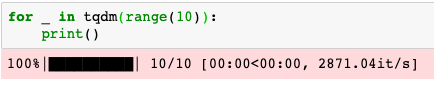So, next time you are just about to set off long-running loop, and you are not just sure how long it will take, just remember this sub-recipe, and use tqdm.

Python is an interpreted language, which is a great advantage for experimenting, but it can be detrimental to speed. There are different ways to compile your Python code, or to use compiled code from Python.

Let's first look at Cython. Cython is an optimizing static compiler for Python, and the programming language compiled by the Cython compiler. The main idea is to write code in a language very similar to Python, and generate C code. This C code can then be compiled as a binary Python extension. SciPy (and NumPy), scikit-learn, and many other libraries have significant parts written in Cython for speed up. You can find out more about Cython on its website at https://cython.org/:

1. You can use the Cython extension for building cython functions in your notebook:
`%load_ext Cython`
`%%cythondef multiply(float x, float y):    return x * y`
1. We can call this function just like any Python function – with the added benefit that it's already compiled:
`multiply(10, 5)  # 50`

This is perhaps not the most useful example of compiling code. For such a small function, the overhead of compilation is too big. You would probably want to compile something that's a bit more complex.

Numba is a JIT compiler for Python (https://numba.pydata.org/). You can often get a speed-up similar to C or Cython using numba and writing idiomatic Python code like the following:

`from numba import jit@jitdef add_numbers(N):    a = 0    for i in range(N):        a += iadd_numbers(10)`

With autotime activated, you should see something like this:

`time: 2.19 s`

So again, the overhead of the compilation is too big to make a meaningful impact. Of course, we'd only see the benefit if it's offset against the compilation. However, if we use this function again, we should see a speedup. Try it out yourself! Once the code is already compiled, the time significantly improves:

`add_numbers(10)`

You should see something like this:

`time: 867 µs`

There are other libraries that provide JIT compilation including TensorFlow, PyTorch, and JAX, that can help you get similar benefits.

The following example comes directly from the JAX documentation, at https://jax.readthedocs.io/en/latest/index.html:

`import jax.numpy as npfrom jax import jitdef slow_f(x):    return x * x + x * 2.0x = np.ones((5000, 5000)) fast_f = jit(slow_f) fast_f(x)`

So there are different ways to get speed benefits from using JIT or ahead-of-time compilation. We'll see some other ways of speeding up your code in the following sections.

# Speeding up pandas DataFrames

One of the most important libraries throughout this book will be pandas, a library for tabular data that's useful for Extract, TransformLoad (ETL) jobs. Pandas is a wonderful library, however; once you get to more demanding tasks, you'll hit some of its limitations. Pandas is the go-to library for loading and transforming data. One problem with data processing is that it can be slow, even if you vectorize the function or if you use df.apply().

You can move further by parallelizing apply. Some libraries, such as swifter, can help you by choosing backends for computations for you, or you can make the choice yourself:

• You can use Dask DataFrames instead of pandas if you want to run on multiple cores of the same or several machines over a network.
• You can use CuPy or cuDF if you want to run computations on the GPU instead of the CPU. These have stable integrations with Dask, so you can run both on multiple cores and multiple GPUs, and you can still rely on a pandas-like syntax (see https://docs.dask.org/en/latest/gpu.html).

As we've mentioned, swifter can choose a backend for you with no change of syntax. Here is a quick setup for using pandas with swifter:

`import pandas as pdimport swifterdf = pd.read_csv('some_big_dataset.csv')df['datacol'] = df['datacol'].swifter.apply(some_long_running_function)`

Generally, apply() is much faster than looping over DataFrames.

You can further improve the speed of execution by using the underlying NumPy arrays directly and accessing NumPy functions, for example, using df.values.apply(). NumPy vectorization can be a breeze, really. See the following example of applying a NumPy vectorization on a pandas DataFrame column:

`squarer = lambda t: t ** 2vfunc = np.vectorize(squarer)df['squared'] = vfunc(df[col].values)`

These are just two ways, but if you look at the next sub-recipe, you should be able to write a parallel map function as yet another alternative.

One way to get something done more quickly is to do multiple things at once. There are different ways to implement your routines or algorithms with parallelism. Python has a lot of libraries that support this functionality. Let's see a few examples with multiprocessing, Ray, joblib, and how to make use of scikit-learn's parallelism.

The multiprocessing library comes as part of Python's standard library. Let's look at it first. We don't provide a dataset of millions of points here – the point is to show a usage pattern – however, please imagine a large dataset. Here's a code snippet of using our pseudo-dataset:

`# run on multiple coresimport multiprocessingdataset = [    {        'data': 'large arrays and pandas DataFrames',        'filename': 'path/to/files/image_1.png'    }, # ... 100,000 datapoints]def get_filename(datapoint):    return datapoint['filename'].split('/')[-1]pool = multiprocessing.Pool(64)result = pool.map(get_filename, dataset)`

Using Ray, you can parallelize over multiple machines in addition to multiple cores, leaving your code virtually unchanged. Ray efficiently handles data through shared memory (and zero-copy serialization) and uses a distributed task scheduler with fault tolerance:

`# run on multiple machines and their coresimport rayray.init(ignore_reinit_error=True)@ray.remotedef get_filename(datapoint):    return datapoint['filename'].split('/')[-1]result = []for datapoint in dataset:    result.append(get_filename.remote(datapoint))`

Scikit-learn, the machine learning library we installed earlier, internally uses joblib for parallelization. The following is an example of this:

`from joblib import Parallel, delayeddef complex_function(x):    '''this is an example for a function that potentially coult take very long.    '''    return sqrt(x)Parallel(n_jobs=2)(delayed(complex_function)(i ** 2) for i in range(10))`

This would give you [0.0, 1.0, 2.0, 3.0, 4.0, 5.0, 6.0, 7.0, 8.0, 9.0]. We took this example from the joblib examples about parallel for loops, available at https://joblib.readthedocs.io/en/latest/parallel.html.

When using scikit-learn, watch out for functions that have an n_jobs parameter. This parameter is directly handed over to joblib.Parallel (https://github.com/joblib/joblib/blob/master/joblib/parallel.py). none (the default setting) means sequential execution, in other words, no parallelism. So if you want to execute code in parallel, make sure to set this n_jobs parameter, for example, to -1 in order to make full use of all your CPUs.

PyTorch and Keras both support multi-GPU and multi-CPU execution. Multi-core parallelization is done by default. Multi-machine execution in Keras is getting easier from release to release with TensorFlow as the default backend.

While notebooks are convenient, they are often messy, not conducive to good coding habits, and they cannot be versioned cleanly. Fastai has developed an extension for literate code development in notebooks called nbdev (https://github.com/fastai/nbdev), which provides tools for exporting and documenting code.

There are a lot more useful extensions that you can find in different places:

We would also like to highlight the following extensions:

Some other libraries used or mentioned in this recipe include the following:

# Classifying in scikit-learn, Keras, and PyTorch

In this section, we'll be looking at data exploration, and modeling in three of the most important libraries. Therefore, we'll break things down into the following sub-recipes:

• Visualizing data in Seaborn
• Modeling in scikit-learn
• Modeling in Keras
• Modeling in PyTorch

Throughout these recipes and several subsequent ones, we'll focus on covering first the basics of the three most important libraries for AI in Python: scikit-learn, Keras, and PyTorch. Through this, we will introduce basic and intermediate techniques in supervised machine learning with deep neural networks and other algorithms. This recipe will cover the basics of these three main libraries in machine learning and deep learning.

We'll go through a simple classification task using scikit-learn, Keras, and PyTorch in turn. We'll run both of the deep learning frameworks in offline mode.

These recipes are for introducing the basics of the three libraries. However, even if you've already worked with all of them, you might still find something of interest.

The Iris Flower dataset is one of the oldest machine learning datasets still in use. It was published by Ronald Fisher in 1936 to illustrate linear discriminant analysis. The problem is to classify one of three iris flower species based on measurements of sepal and petal width and length.

Although this is a very simple problem, the basic workflow is as follows:

2. Visualize the data.
3. Preprocess and transform the data.
4. Choose a model to use.
5. Check the model performance.
6. Interpret and understand the model (this stage is often optional).

This is a standard process template that we will have to apply to most of the problems shown throughout this book. Typically, with industrial-scale problems, Steps 1 and 2 can take much longer (sometimes estimated to take about 95 percent of the time) than for one of the already preprocessed datasets that you will get for a Kaggle competition or at the UCI machine learning repository. We will go into the complexities of each of these steps in later recipes and chapters.

We'll assume you've installed the three libraries earlier on and that you have your Jupyter Notebook or Colab instance running. Additionally, we will use the seaborn and scikit-plot libraries for visualization, so we'll install them as well:

`!pip install seaborn scikit-plot`

The convenience of using a dataset so well known is that we can easily load it from many packages, for example, like this:

`import seaborn as snsiris = sns.load_dataset('iris')`

Let's jump right in, starting with data visualization.

# How to do it...

Let's first have a look at the dataset.

# Visualizing data in seaborn

In this recipe, we'll go through the basic steps of data exploration. This is often important to understand the complexity of the problem and any underlying issues with the data:

1. Plot a pair-plot:
`%matplotlib inline# this^ is not necessary on Colabimport seaborn as snssns.set(style="ticks", color_codes=True)g = sns.pairplot(iris, hue='species')`

Here it comes (rendered in seaborn's pleasant spacing and coloring):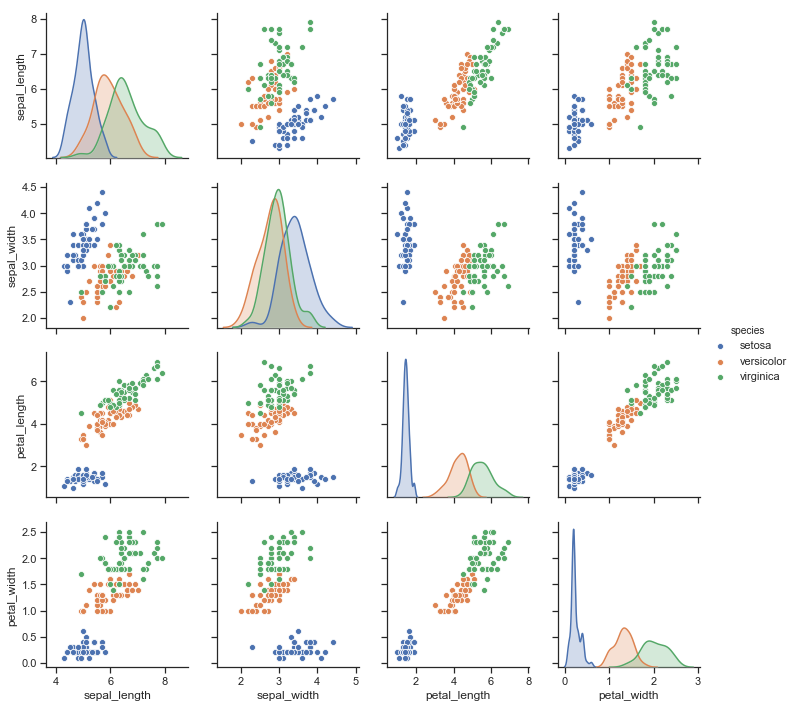A pair-plot in seaborn visualizes pair-wise relationships in a dataset. Each subplot shows one variable plotted against another in a scatterplot. The subplots on the diagonal show the distribution of the variables. The colors correspond to the three classes.

From this plot, especially if you look along the diagonal, we can see that the virginica and versicolor species are not (linearly) separable. This is something we are going to struggle with, and that we'll have to overcome.

1. Let's have a quick look at the dataset:
`iris.head()`

We only see setosa, since the flower species are ordered and listed one after another: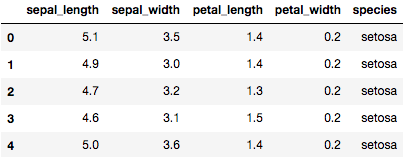1. Separate the features and target in preparation for training as follows:
`classes = {'setosa': 0, 'versicolor': 1, 'virginica': 2}X = iris[['sepal_length', 'sepal_width', 'petal_length', 'petal_width']].valuesy = iris['species'].apply(lambda x: classes[x]).values`

The last line converted the three strings corresponding to the three classes into numbers – this is called an ordinal coding. A multiclass machine learning algorithm can deal with this. For neural networks, we'll use another encoding, as you'll see later.

After these basic steps, we are ready to start developing predictive models. These are models that predict the flower class from the features. We'll see this in turn for each of the three most important machine learning libraries in Python. Let's start with scikit-learn.

# Modeling in scikit-learn

In this recipe, we'll create a classifier in scikit-learn, and check its performance.

Scikit-learn (also known as sklearn) is a Python machine learning framework developed since 2007. It is also one of the most comprehensive frameworks available, and it is interoperable with the pandas, NumPy, SciPy, and Matplotlib libraries. Much of scikit-learn has been optimized for speed and efficiency in Cython, C, and C++.

Please note that not all scikit-learn classifiers can do multiclass problems. All classifiers can do binary classification, but not all can do more than two classes. The random forest model can, fortunately. The random forest model (sometimes referred to as random decision forest) is an algorithm that can be applied to classification and regression tasks, and is an ensemble of decision trees. The main idea is that we can increase precision by creating decision trees on bootstrapped samples of the dataset, and average over these trees.

Some of the following lines of code should appear to you as boilerplate, and we'll use them over and over:

1. Separate training and validation.

As a matter of good practice, we should always test the performance of our models on a sample of our data that wasn't used in training (referred to as a hold-out set or validation set). We can do this as follows:

`from sklearn.ensemble import RandomForestClassifierfrom sklearn.model_selection import train_test_splitX_train, X_test, y_train, y_test = train_test_split(    X, y, test_size=0.33, random_state=0)`
1. Define a model.

Here we define our model hyperparameters, and create the model instance with these hyperparameters. This goes as follows in our case:

Hyperparameters are parameters that are not part of the learning process, but control the learning. In the case of neural networks, this includes the learning rate, model architecture, and activation functions.
`params = dict(    max_depth=20,    random_state=0,    n_estimators=100,)clf = RandomForestClassifier(**params)`
1. Train the model.

Here, we pass the training dataset to our model. During training, the parameters of the model are being fit so that we obtain better results (where better is defined by a function, called the cost function or loss function).

For training we use the fit method, which is available for all sklearn-compatible models:

`clf.fit(X_train, y_train)`
1. Check the performance of the model.

While there's a measure internal to the model (the cost function), we might want to look at additional measures. In the context of modeling, these are referred to as metrics. In scikit-learn, we have a lot of metrics at our fingertips. For classification, we would usually look at the confusion matrix, and often we'd want to plot it:

`from sklearn.metrics import plot_confusion_matrixplot_confusion_matrix(    clf, X_test, y_test,    display_labels=['setosa', 'versicolor', 'virginica'],    normalize='true')`

The confusion matrix is relatively intuitive, especially when the presentation is as clear as with sklearn's plot_confusion_matrix(). Basically, we see how well our class predictions line up with the actual classes. We can see the predictions against actual labels, grouped by class, so that each entry corresponds to how many times class A was predicted given the actual class B. In this case, we've normalized the matrix, so that each row (actual labels) sums to one.

Here is the confusion matrix: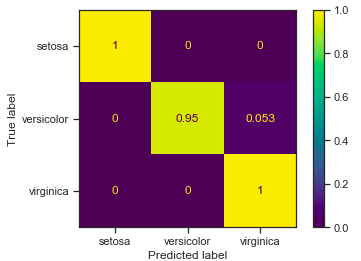Since this is a normalized martix, the numbers on the diagonal are also called the hit rate or true positive rateWe can see that setosa was predicted as setosa 100% (1) of the time. By contrast, versicolor was predicted as versicolor 95% of the time (0.95), while 5% of the time (0.053) it was predicted as virginica.

The performance is very good in terms of hit rate, however; as expected, we are having a small problem distinguishing between versicolor and virginica.

Let's move on to Keras.

# Modeling in Keras

In this recipe, we'll be predicting the flower species in Keras.

Keras is a high-level interface for (deep) neural network models that can use TensorFlow as a backend, but also Microsoft Cognitive Toolkit (CNTK), Theano, or PlaidML. Keras is an interface for developing AI models, rather than a standalone framework itself. Keras has been integrated as part of TensorFlow, so we import Keras from TensorFlow. Both TensorFlow and Keras are open source and developed by Google.

Since Keras is tightly integrated with TensorFlow, Keras models can be saved as TensorFlow models and then deployed in Google's deployment system, TensorFlow Serving (see https://www.tensorflow.org/tfx/guide/serving), or used from any of the programming languages such as, C++ or Java. Let's get into it:

1. Run the following code. If you are familiar with Keras, you'll recognize it as boilerplate:
`from tensorflow.keras.models import Sequentialfrom tensorflow.keras.layers import Denseimport tensorflow as tfdef create_iris_model():    '''    Create the iris classification model    '''    iris_model = Sequential()    iris_model.add(Dense(10, activation='selu', input_dim=4))    iris_model.add(Dense(3, activation='softmax'))    iris_model.compile(        optimizer='rmsprop',        loss='categorical_crossentropy',        metrics=['accuracy']    )    iris_model.summary()    return iris_modeliris_model = create_iris_model()`

This yields the following model construction: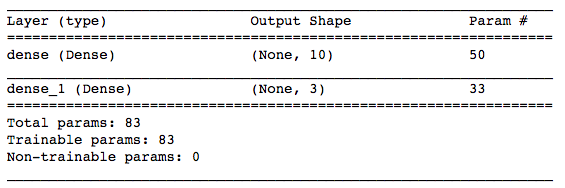We can visualize this model in different ways. We can use the built-in Keras functionality as follows:

`dot = tf.keras.utils.model_to_dot(    iris_model,    show_shapes=True,    show_layer_names=True,    rankdir="TB",    expand_nested=True,    dpi=96,    subgraph=False,)dot.write_png('iris_model_keras.png')`

This writes a visualization of the network to a file called iris_model_keras.png. The image produced looks like this: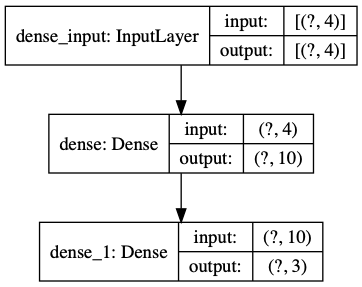This shows that we have 4 input neurons, 10 hidden neurons, and 3 output neurons, fully connected in a feed-forward fashion. This means that all neurons in the input feed input to all neurons in the hidden layer, which in turn feed to all neurons in the output layer.

We are using the sequential model construction (as opposed to the graph). The sequential model type is more straightforward to build than the graph type. The layers are constructed the same way; however, for the sequential model, you have to define the input dimensionality, input_dim.

We use two dense layers, the intermediate layer with SELU activation function, and the final layer with the softmax activation function. We'll explain both of these in the How it works... section. As for the SELU activation function, suffice it to say for now that it provides a necessary nonlinearity so that the neural network can deal with more variables that are not linearly separable, as in our case. In practice, it is rare to use a linear (identity function) activation in the hidden layers.

Each unit (or neuron) in the final layer corresponds to one of the three classes. The softmax function normalizes the output layer so that its neural activations add up to 1. We train with categorical cross-entropy as our loss function. Cross-entropy is typically used for classification problems with neural networks. The binary cross-entropy loss is for two classes, and categorical cross-entropy is for two or more classes (cross-entropy will be explained in more detail in the How it works... section).

1. Next, one-hot encode the features.

This means we have three columns that each stand for one of the species, and one of them will be set to 1 for the corresponding class:

`y_categorical = tf.keras.utils.to_categorical(y, 3)`

Our y_categorical therefore has the shape (150, 3). This means that to indicate class 0 as the label, instead of having a 0 (this would be sometimes called label encoding or integer encoding), we have a vector of [1.0, 0.0, 0.0]. This is called one-hot encodingThe sum of each row is equal to 1.

1. Normalize the features.

For neural networks, our features should be normalized in a way that the activation functions can deal with the whole range of inputs – often this normalization is to the standard distribution, which has a mean of 0.0 and standard deviation of 1.0:

`X = (X - X.mean(axis=0)) / X.std(axis=0)X.mean(axis=0)`

The output of this cell is this:

`array([-4.73695157e-16, -7.81597009e-16, -4.26325641e-16, -4.73695157e-16])`

We see that the mean values for each column are very close to zero. We can also see the standard deviations with the following command:

`X.std(axis=0)`

The output is as follows:

`array([1., 1., 1., 1.])`

The standard deviation is exactly 1, as expected.

1. Display our training progress in TensorBoard.

TensorBoard is a visualization tool for neural network learning, such as tracking and visualizing metrics, model graphs, feature histograms, projecting embeddings, and much more:

`%load_ext tensorboardimport oslogs_base_dir = "./logs"os.makedirs(logs_base_dir, exist_ok=True)%tensorboard --logdir {logs_base_dir}`

At this point, a TensorBoard widget should pop up in your notebook. We just have to make sure it gets the information it needs:

1. Plug the TensorBoard details into the Keras training function as a callback so TensorBoard gets the training information:
`import datetime  logdir = os.path.join(  logs_base_dir,  datetime.datetime.now().strftime("%Y%m%d-%H%M%S"))tensorboard_callback = tf.keras.callbacks.TensorBoard(  logdir, histogram_freq=1)X_train, X_test, y_train, y_test = train_test_split(    X, y_categorical, test_size=0.33, random_state=0)iris_model.fit(  x=X_train,  y=y_train,  epochs=150,  callbacks=[tensorboard_callback])`

This runs our training. An epoch is an entire pass of the dataset through the neural network. We use 150 here, which is a bit arbitrary. We could have used a stopping criterion to stop training automatically when validation and training errors start to diverge, or in other words, when overfitting occurs.

In order to use plot_confusion_matrix() as before, for comparison, we'd have to wrap the model in a class that implements the predict() method, and has a list of classes_ and an attribute of _estimator_type that is equal to the classifier. We will show that in the online material.

1. Plot the confusion matrix.

Here, it's easier to use a scikitplot function:

`import scikitplot as skplty_pred = iris_model.predict(X_test).argmax(axis=1)skplt.metrics.plot_confusion_matrix(    y_test.argmax(axis=1),    y_pred,    normalize=True)`

Again, as before, we normalize the matrix, so we get fractions. The output should look similar to the following: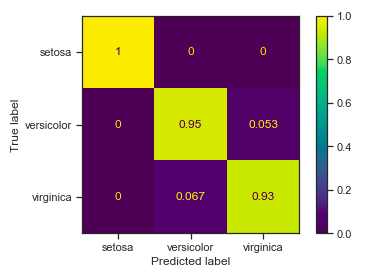This is a bit worse than our previous attempt in scikit-learn, but with some tweaking we can get to a comparable level, or maybe even better performance. Examples of tweaking would be changing any of the model's hyperparameters such as the number of neurons in the hidden layer, any changes to the network architecture (adding a new layer), or changing the activation function of the hidden layer.

1. Check the charts from TensorBoard: the training progress and the model graph. Here they are: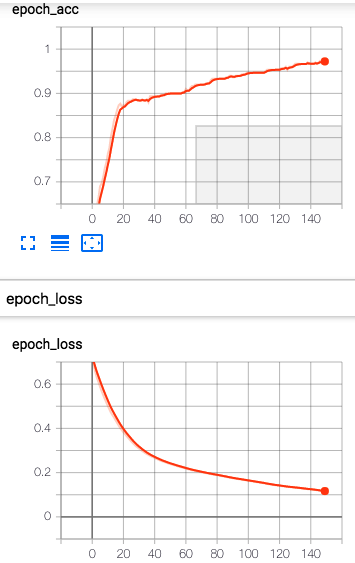These plots show the accuracy and loss, respectively, over the entire training. We also get another visualization of the network in TensorBoard: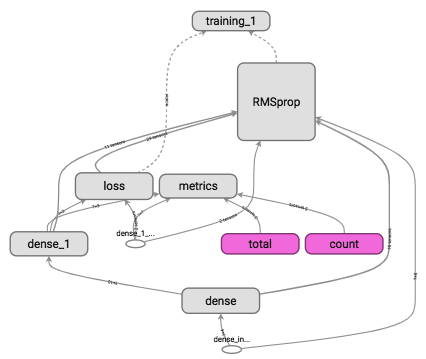This shows all the network layers, the loss and metrics, the optimizer (RMSprop), and the training routine, and how they are related. As for the network architecture, we can see four dense layers (the presented input and targets are not considered proper parts of the network, and are therefore colored in white). The network consists of a dense hidden layer (being fed by the input), and an dense output layer (being fed by the hidden layer). The loss function is calculated between the output layer activation and the targets. The optimizer works with all layers based on the loss. You can find a tutorial on TensorBoard at https://www.tensorflow.org/tensorboard/get_started. The TensorBoard documentation explains more about configuration and options.

So the classification accuracy is improving and the loss is decreasing over the course of the training epochs. The final graph shows the network and training architecture, including the two dense layers, the loss and metrics, and the optimizer.

# Modeling in PyTorch

In this recipe, we will describe a network equivalent to the previous one shown in Keras, train it, and plot the performance.

PyTorch is a deep learning framework that is based on the Torch library primarily developed by Facebook. For some time, Facebook was developing another deep learning framework, called Caffe2; however, it was merged into PyTorch in March 2018. Some of the strengths of PyTorch are in image and language processing applications. Apart from Python, Torch provides a C++ interface, both for learning and model deployment:

1. Let's define the model architecture first. This looks very similar to Keras:
`import torchfrom torch import nniris_model = nn.Sequential(  torch.nn.Linear(4, 10), # equivalent to Dense in keras  torch.nn.SELU(),  torch.nn.Linear(10, 3),  torch.nn.Softmax(dim=1))print(iris_model)`

This is the same architecture that we defined before in Keras: this is a feed-forward, two-layer neural network with a SELU activation on the hidden layer, and 10 and 3 neurons in the 2 layers.

If you prefer an output similar to the summary() function in Keras, you can use the torchsummary package (https://github.com/sksq96/pytorch-summary).
1. We need to convert our NumPy arrays to Torch tensors:
`from torch.autograd import VariableX_train = Variable(    torch.Tensor(X_train).float())y_train = Variable(torch.Tensor(   y_train.argmax(axis=1)).long())X_test = Variable(    torch.Tensor(X_test).float())y_test = Variable(    torch.Tensor(y_test.argmax(axis=1)).long())`

y_train is the one-hot encoded target matrix that we created earlier. We are converting it back to integer encoding since the PyTorch cross-entropy loss expects this.

1. Now we can train, as follows:
```criterion = torch.nn.CrossEntropyLoss()  # cross entropy loss
optimizer = torch.optim.RMSprop(    iris_model.parameters(), lr=0.01)for epoch in range(1000):  optimizer.zero_grad()  out = iris_model(X_train)  loss = criterion(out, y_train)  loss.backward()  optimizer.step()  if epoch % 10 == 0:    print('number of epoch', epoch, 'loss', loss)```
1. And then we'll use scikitplot to visualize our results, similar to before:
`import scikitplot as skplty_pred = iris_model(X_test).detach().numpy()skplt.metrics.plot_confusion_matrix(    y_test,    y_pred.argmax(axis=1),    normalize=True)labels = ['setosa', 'versicolor', 'virginica']ax.set_xticklabels(labels)ax.set_yticklabels(labels)`

This is the plot we get: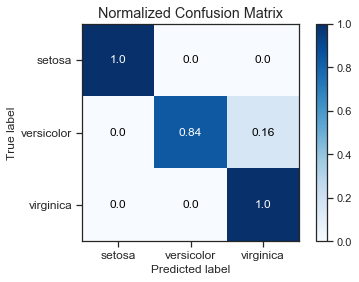Your plot might differ. Neural network learning is not deterministic, so you could get better or worse numbers, or just different ones.

We can get better performance if we let this run longer. This is left as an exercise for you.

# How it works...

We'll first look at the intuitions behind neural network training, then we'll look a bit more at some of the technical details that we will use in the PyTorch and Keras recipes.

# Neural network training

The basic idea in machine learning is that we try to minimize an error by changing the parameters of a model. This adaption of the parameter is called learning. In supervised learning, the error is defined by a loss function calculated between the prediction of the model and the target. This error is calculated at every step and the model parameters are adjusted accordingly.

Neural networks are composable function approximators consisting of tunable affine transformations (f) with an activation function (sigma):In the simplest terms, in a feed-forward neural network of one layer with linear activations, the model predictions are given by the sum of the product of the coefficients with the input in all of its dimensions:This is called a perceptron, and it is a linear binary classifier. A simple illustration with four inputs is shown in the following diagram: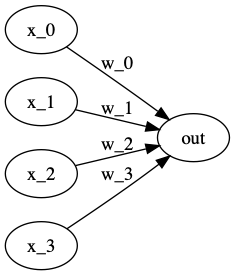The predictor for a one-dimensional input breaks down to the slope-intercept form of a line in two dimensions,. Here, m is the slope and b the y-intercept. For higher-dimensional inputs, we can write (changing notation and vectorizing)with bias termand weights. This is still a line, just in a space of the same dimensionality as the input. Please note thatdenotes our model prediction for, and for the examples where we know, we can calculate the difference between the two as our prediction error.

We can also use the same very simple linear algebra to define the binary classifier by thresholding as follows:This is still very simple linear algebra. This linear model with just one layer, called a perceptron, has difficulty predicting any more complex relationships. This lead to deep concern about the limitations of neural networks following an influential paper by Minsky and Papert in 1969. However, since the 1990s, neural networks have been experiencing a resurgence in the shape of support vector machines (SVMs) and the multilayer perceptron (MLP). The MLP is a feed-forward neural network with at least one layer between the input and output (hidden layer). Since a multilayer perceptron with many layers of linear activations can be reduced to just one layer, non-trivially, we'll be referring to neural networks with hidden layers and nonlinear activation functions. These types of models can approximate arbitrary functions and perform nonlinear classification (according to the Universal Approximation Theorem). The activation function on any layer can be any differentiable nonlinearity; traditionally, the sigmoid,, has been used a lot for this purpose.

For illustration, let's write this down with jax:

`import jax.numpy as npfrom jax import grad, jitimport numpy.random as nprdef predict(params, inputs):    for W, b in params:        outputs = np.dot(inputs, W) + b        inputs = np.tanh(outputs)    return outputsdef construct_network(layer_sizes=[10, 5, 1]):    '''Please make sure your final layer corresponds to    the target dimensionality.    '''    def init_layer(n_in, n_out):        W = npr.randn(n_in, n_out)        b = npr.randn(n_out,)        return W, b    return list(        map(init_layer, layer_sizes[:-1], layer_sizes[1:])    )params = construct_network()`

If you look at this code, you'll see that we could have equally written this up with operations in NumPy, TensorFlow, or PyTorch. You'll also note that the construct_network() function takes a layer_sizes argument. This is one of the hyperparameters of the network, something to decide on before learning. We can choose just an output of  to get the perceptron, or [10, 1] to get a two-layer perceptron. So this shows how to get a network as a set of parameters and how to get a prediction from this network. We still haven't discussed how we learn the parameters, and this brings us to errors.

There's an adage that says, "all models are wrong, but some are useful." We can measure the error of our model, and this can help us to calculate the magnitude and direction of changes that we can make to our parameters in order to reduce the error.

Given a (differentiable) loss function (also called the cost function),, such as the mean squared error (MSE), we can calculate our error. In the case of the MSE, the loss function is as follows:Then in order to get the change to our weights, we'll use the derivative of the loss over the points in training:This means we are applying a gradient descent, which means that over time, our error will be reduced proportionally to the gradient (scaled by learning rate). Let's continue with our code:

`def mse(preds, targets):    return np.sum((preds - targets)**2)def propagate_and_error(loss_fun):    def error(params, inputs, targets):        preds = predict(params, inputs)        return loss_fun(preds, targets)    return errorerror_grads = jit(grad(propagate_and_error(mse)))`

Both PyTorch and JAX have autograd functionality, which means that we can automatically get derivatives (gradients) of a wide range of functions.

We'll encounter a lot of different activation and loss functions throughout this book. In this chapter, we used the SELU activation function.

# The SELU activation function

The scaled exponential linear unit (SELU) activation function was published quite recently by Klambauer et al in 2017 (http://papers.nips.cc/paper/6698-self-normalizing-neural-networks.pdf):The SELU function is linear for positive values of x, a scaled exponential for negative values, and 0 when x is 0.is a value greater than 1.  You can find the details in the original paper. The SELU function has been shown to have better convergence properties than other functions. You can find a comparison of activation functions in Padamonti (2018) at https://arxiv.org/pdf/1804.02763.pdf.

# Softmax activation

As our activation function for the output layer in the neural networks, we use a softmax function. This works as a normalization to sum 1.0 of the neural activations of the output layer. The output can be therefore interpreted as the class probabilities. The softmax activation function is defined as follows:# Cross-entropy

In the multiclass training with neural networks, it's common to train for cross-entropy. The binary cross-entropy for multiclass cases looks like the following:Here, M is the number of classes (setosa, versicolor, and virginica), y is 0 or 1 if the class label c is correct, and p is the predicted probability that the observation o is of class c. You can read up more on different loss functions and metrics on the ml-cheatsheet site, at https://ml-cheatsheet.readthedocs.io/en/latest/loss_functions.html.

You can find out more details on the website of each of the libraries used in this recipe:

TensorboardX is a TensorBoard interface for other deep learning frameworks apart from TensorFlow (PyTorch, Chainer, MXNet, and others), available at https://github.com/lanpa/tensorboardX.

It should probably be noted that scikit-plot is not maintained anymore. For the plotting of machine learning metrics and charts, mlxtend is a good option, at http://rasbt.github.io/mlxtend/.

Some other libraries we used here and that we will encounter throughout this book include the following:

In the following recipe, we'll get to grips with a more realistic example in Keras.

# Modeling with Keras

In this recipe, we will load a dataset and then we will conduct exploratory data analysis (EDA), such as visualizing the distributions.

We will do typical preprocessing tasks such as encoding categorical variables, and normalizing and rescaling for neural network training. We will then create a simple neural network model in Keras, train the model plotting using a generator, and plot the training and validation performance. We will look at a still quite simple dataset: the dult dataset from the UCI machine learning repository. With this dataset (also known as the Census Income dataset), the goal is to predict from census data whether someone earns more than US\$50,000 per year.

Since we have a few categorical variables, we'll also deal with the encoding of categorical variables.

Since this is still an introductory recipe, we'll go through this problem with a lot of detail for illustration. We'll have the following parts:

2. Inspecting the data
3. Categorical encoding
4. Plotting variables and distributions
5. Plotting correlations
6. Label encoding
7. Normalizing and scaling
8. Saving the preprocessed data
• Model training:

1. Creating the model
2. Writing a data generator
3. Training the model
4. Plotting the performance
5. Extracting performance metrics
6. Calculating feature importances

We'll need a few libraries for this recipe in addition to the libraries we installed earlier:

• category_encoders for the encoding of categorical variables
• minepy for information-based correlation measures
• eli5 for the inspection of black-box models

We've used Seaborn before for visualization.

We can install these libraries as follows:

`!pip install category_encoders minepy eli5 seaborn`
As a note to you, the reader: if you use pip and conda together, there is a danger that some of the libraries might become incompatible, creating a broken environment. We'd recommend using conda when a version of conda is available, although it is usually faster to use pip

This dataset is already split into training and test. Let's download the dataset from UCI as follows:

`!wget http://archive.ics.uci.edu/ml/machine-learning-databases/adult/adult.data!wget http://archive.ics.uci.edu/ml/machine-learning-databases/adult/adult.test`

wget doesn't ship with macOS by default; we suggest installing wget using brew (https://formulae.brew.sh/formula/wget). On Windows, you can visit the two preceding URLs and download both via the File menu. Make sure you remember the directory where you save the files, so you can find them later. There are a few alternatives, however:

• You can use the download script we provide in Chapter 2, Advanced Topics in Supervised Machine Learning, in the Predicting house prices in PyTorch recipe.
• You can install the wget library and run import wget; wget.download(URL, filepath).
We have the following information from the UCI dataset description page:
- age: continuous.
- workclass: Private, Self-emp-not-inc, Self-emp-inc, Federal-gov, Local-gov, State-gov, Without-pay, Never-worked.
- fnlwgt: continuous.
- education: Bachelors, Some-college, 11th, HS-grad, Prof-school, Assoc-acdm, Assoc-voc, 9th, 7th-th, 12th, Masters, 1st-4th, 10th, Doctorate, 5th-6th, Preschool.
- education-num: continuous.
- marital-status: Married-civ-spouse, Divorced, Never-married, Separated, Widowed, Married-spouse-absent, Married-AF-spouse.
- occupation: Tech-support, Craft-repair, Other-service, Sales, Exec-managerial, Prof-specialty, Handlers-cleaners, Machine-op-inspct, Adm-clerical, Farming-fishing, Transport-moving, Priv-house-serv, Protective-serv, Armed-Forces.
- relationship: Wife, Own-child, Husband, Not-in-family, Other-relative, Unmarried.
- race: White, Asian-Pac-Islander, Amer-Indian-Eskimo, Other, Black.
- sex: Female, Male.
- capital-gain: continuous.
- capital-loss: continuous.
- hours-per-week: continuous.
- native-country: United-States, and so on.

fnlwgt actually stands for the final weight; in other words, the total number of people constituting the entry.

Please keep in mind that this dataset is a well-known dataset that has been used many times in scientific publications and in machine learning tutorials. We are using it here to go over some basics in Keras without having to focus on the dataset.

# How to do it...

As we've mentioned before, we'll first load the dataset, do some EDA, then create a model in Keras, train it, and look at the performance.

We've split this recipe up into data loading and preprocessing, and secondly, model training.

`import pandas as pdcols = [    'age', 'workclass', 'fnlwgt',    'education', 'education-num',    'marital-status', 'occupation',    'relationship', 'race', 'sex',    'capital-gain', 'capital-loss',    'hours-per-week', 'native-country', '50k']train = pd.read_csv(    'adult.data',    names=cols)test = pd.read_csv(    'adult.test',    names=cols)`

Now let's look at the data!

1. Inspecting the data: The beginning of the DataFrame we can see with the head() method:
`train.head()`

This yields the following output: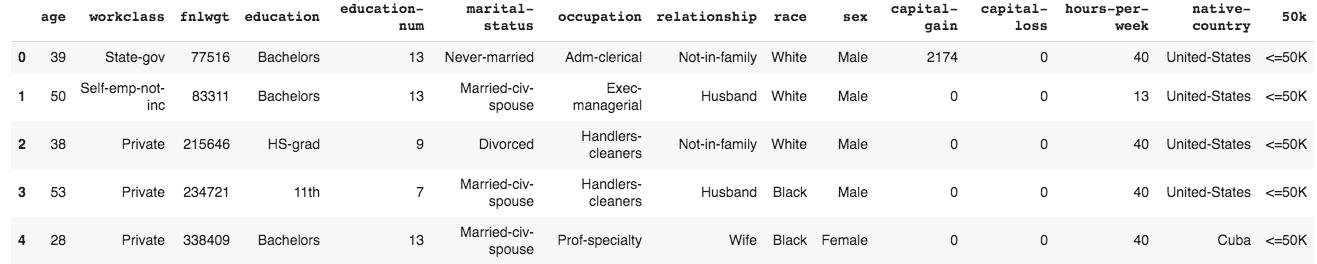Next, we'll look at the test data:

`test.head()`

This looks as follows: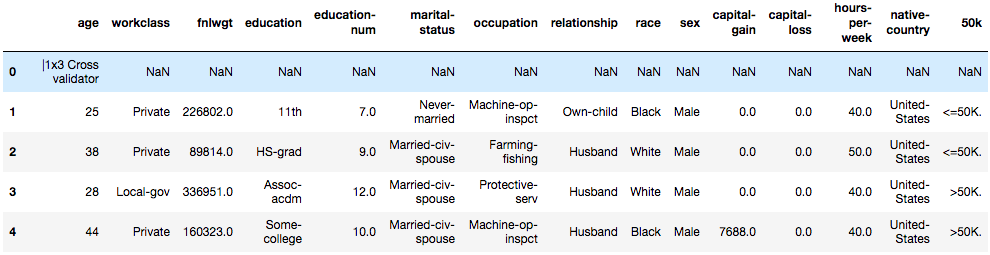The first row has 14 nulls and 1 unusable column out of 15 columns. We will discard this row:

`test.drop(0, axis=0, inplace=True)`

And it's gone.

1. Categorical encoding: Let's start with category encoding. For EDA, it's good to use ordinal encoding. This means that for a categorical feature, we map each value to a distinct number:
`import category_encoders as ceX = train.drop('50k', axis=1)encoder = ce.OrdinalEncoder(cols=list(    X.select_dtypes(include='object').columns)[:])encoder.fit(X, train['50k'])X_cleaned = encoder.transform(X)X_cleaned.head()`

We are separating X, the features, and y, the targets, here. The features don't contain the labels; that's the purpose of the drop() method – we could have equally used del train['50k'].

Here is the result: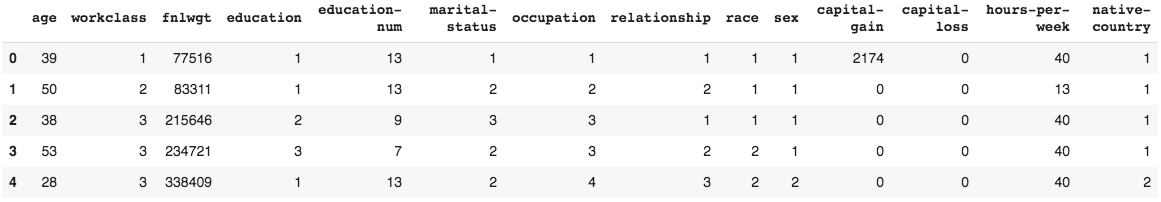When starting with a new task, it's best to do EDA. Let's plot some of these variables.

1. To plot variables and distributions, use the following code block:
`from scipy import statsimport seaborn as snssns.set(color_codes=True)sns.set_context( 'notebook', font_scale=1.5, rc={"lines.linewidth": 2.0})sns.distplot(train['age'], bins=20, kde=False, fit=stats.gamma)`

We'll get the following plot: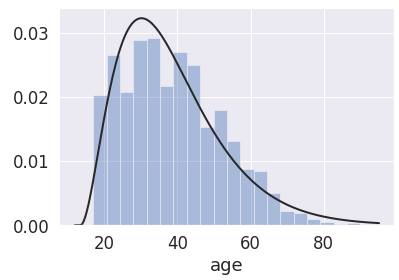Next, we'll look at a pair-plot again. We'll plot all numerical variables against each other:

`import numpy as npnum_cols = list(  set(    train.select_dtypes(      include='number'    ).columns  ) - set(['education-num'])) + ['50k']]g = sns.pairplot( train[num_cols], hue='50k', height=2.5, aspect=1,)for i, j in zip(*np.triu_indices_from(g.axes, 1)):  g.axes[i, j].set_visible(False)`

As discussed in the previous recipe, the diagonal in the pair-plot shows us histograms of single variables – that is, the distribution of the variable – with the hue defined by the classes. Here we have orange versus blue (see the legend on the right of the following plot). The following subplots on the diagonal show scatter plots between the two variables: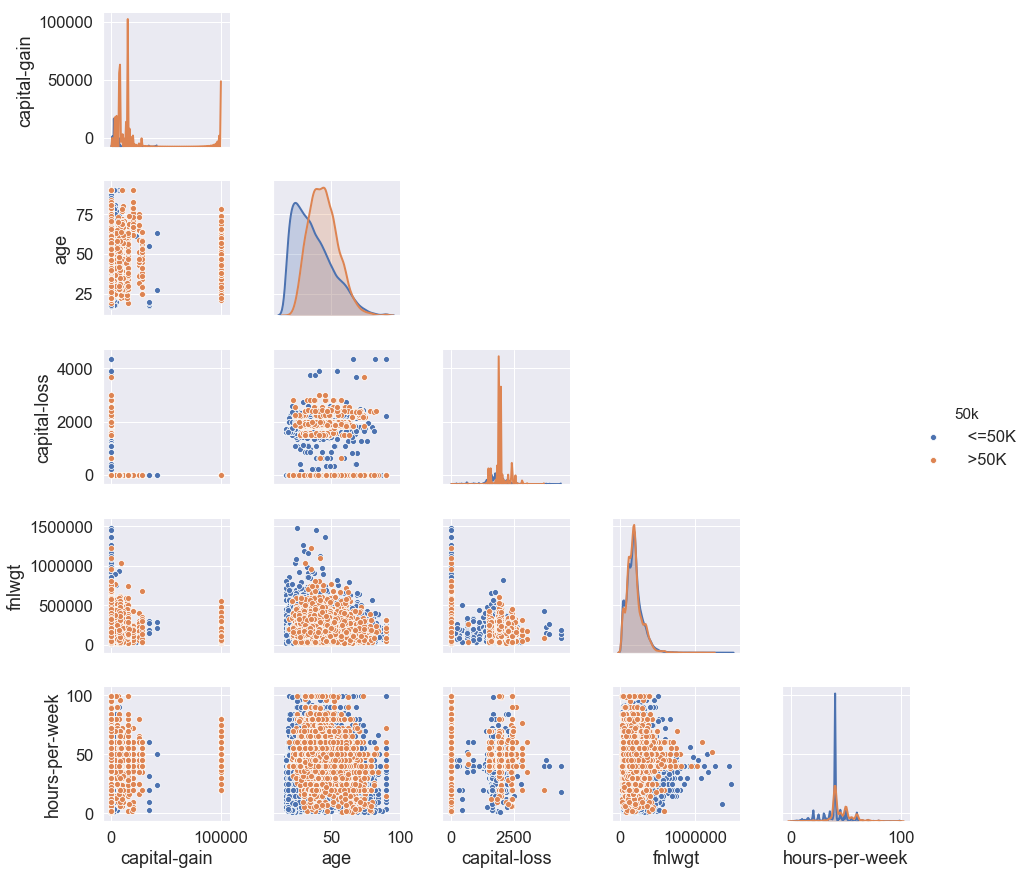If we look at the age variable on the diagonal (second row), we see that the two classes have a different distribution, although they are still overlapping. Therefore, age seems to be discriminative with respect to our target class.

We can see that in a categorical plot as well:

`sns.catplot(x='50k', y='age', kind='box', data=train)`

Here's the resulting plot: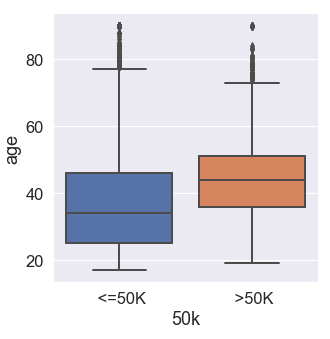After this, let's move on to a correlation plot.

1. Plotting correlations: In order to get an idea of the redundancy between variables, we'll plot a correlation matrix based on the Maximal Information Coefficient (MIC), a correlation metric based on information entropy. We'll explain the MIC at the end of this recipe.

Since the MIC can take a while to compute, we'll take the parallelization pattern we introduced   earlier. Please note the creation of the thread pool and the map operation:

`import numpy as npimport osfrom sklearn.metrics.cluster import adjusted_mutual_info_scorefrom minepy import MINEimport multiprocessingdef calc_mic(args):  (a, b, i1, i2) = args  mine = MINE(alpha=0.6, c=15, est='mic_approx')  mine.compute_score(a, b)  return (mine.mic(), i1, i2)pool = multiprocessing.Pool(os.cpu_count())corrs = np.zeros((len(X_cleaned.columns), len(X_cleaned.columns)))queue = []for i1, col1 in enumerate(X_cleaned.columns):  if i1 == 1:    continue  for i2, col2 in enumerate(X_cleaned.columns):    if i1 < i2:      continue    queue.append((X_cleaned[col1], X_cleaned[col2], i1, i2))results = pool.map(calc_mic, queue)for (mic, i1, i2) in results:  corrs[i1, i2] = miccorrs = pd.DataFrame(    corrs,    columns=list(X_cleaned.columns),    index=list(X_cleaned.columns))`

This can still take a while, but should be much faster than doing the computations in sequence.

Let's visualize the correlation matrix as a heatmap: since the matrix is symmetric, here, we'll only show the lower triangle and apply some nice styling:

`mask = np.zeros_like(corrs, dtype=np.bool)mask[np.triu_indices_from(mask)] = Truecmap = sns.diverging_palette(    h_neg=220, h_pos=10, n=50, as_cmap=True)sns.set_context(    'notebook', font_scale=1.1,    rc={'lines.linewidth': 2.0})sns.heatmap(    corrs,    square=True,    mask=mask,    cmap=cmap, vmax=1.0, center=0.5,    linewidths=.5,    cbar_kws={"shrink": .5})`

This looks as follows: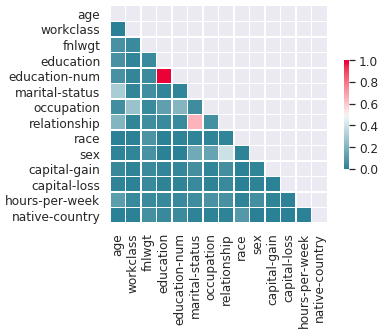We can see in the correlation matrix heatmap that most pair correlations are pretty low (most correlations are below 0.4), meaning that most features are relatively uncorrelated; however, there is one pair of variables that stands out, those of education-num and education:

`corrs.loc['education-num', 'education']`

The output is 0.9995095286140694.

This is about as close to a perfect correlation as it can get. These two variables do in fact refer to the same information.

Let's see the variance in education-num for each value in education:

`train.groupby(by='education')['education-num'].std()`

We only see zeros. There's no variance. In other words, each value in education corresponds to exactly one value in education-num. The variables are exactly the same! We should be able to remove one of them, for example with del train['education'], or just ignore one of them during training.

The UCI description page mentions missing variables. Let's look for missing variables now:

`train.isnull().any()`

We only see False for each variable, so we cannot see any missing values here.

In neural network training, for categorical variables, we have the choice of either using embeddings (we'll get to these in Chapter 10, Natural Language Processing) or feeding them as one-hot encodings; this means that each factor, each possible value, is encoded in a binary variable that indicates whether it is given or not. Let's try one-hot encodings for simplicity.

So, first, let's re-encode the variables:

`encoder = ce.OneHotEncoder(    cols=list(X.select_dtypes(include='object').columns)[:])encoder.fit(X, train['50k'])X_cleaned = encoder.transform(X)x_cleaned_cols = X_cleaned.columnsx_cleaned_cols`

Our x_cleaned_cols looks as follows: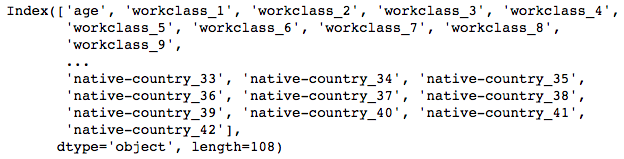After this, it's time to encode our labels.

1. Label encoding: We are going to encode target values in two columns as 1 if present and 0 if not present. It is good to remember that the Python truth values correspond to 0 and 1, respectively, for false and true. Since we have a binary classification task (that is, we only have two classes), we can use 0 and 1 in a single output. If we have more than two classes, we'd have to use categorical encoding for the output, which typically means we use as many output neurons as we do classes. Often, we have to try different solutions in order to see what works best.

In the following code block, we just made a choice and stuck with it:

`y = np.zeros((len(X_cleaned), 2))y[:, 0] = train['50k'].apply(lambda x: x == ' <=50K')y[:, 1] = train['50k'].apply(lambda x: x == ' >50K')`

1. Normalizing and scaling: We have to convert all values to z-values. This is when we subtract the mean and divide by the standard deviation, in order to get a normal distribution with a mean of 0.0 and a standard deviation of 1.0. It's not necessary to have normal distributions for neural network input. However, it's important that numerical values are scaled to the sensitive part of the neural network activation functions. Converting to z-scores is a standard way to do this:

`from sklearn.preprocessing import StandardScalerstandard_scaler = StandardScaler()X_cleaned = standard_scaler.fit_transform(X_cleaned)X_test = standard_scaler.transform(encoder.transform(test[cols[:-1]]))`
1. Saving our preprocessing: For good practice, we save our datasets and the transformers so we have an audit trail. This can be useful for bigger projects:

`import joblibjoblib.dump(    [encoder, standard_scaler, X_cleaned, X_test],    'adult_encoder.joblib')`

We are ready to train now.

# Model training

We'll create the model, train it, plot performance, and then calculate the feature importance.

1. To create the model, we use the Sequential model type again. Here's our network architecture:
`from tensorflow.keras import Sequentialfrom tensorflow.keras.layers import Densemodel = Sequential()model.add(Dense(20, activation='selu', input_dim=108))model.add(Dense(2, activation='softmax'))model.compile(    optimizer='rmsprop',    loss='categorical_hinge',    metrics=['accuracy'])model.summary()`

Here's the Keras model summary: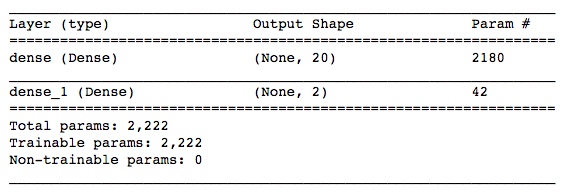1. Now, let's write a data generator. To make this a bit more interesting, we will use a generator this time to feed in our data in batches. This means that we stream in our data instead of putting all of our training data into the fit() function at once. This can be useful for very big datasets.

We'll use the fit_generator() function as follows:

`def adult_feed(X_cleaned, y, batch_size=10, shuffle=True):  def init_batches():    return (        np.zeros((batch_size, X_cleaned.shape)),        np.zeros((batch_size, y.shape))        )  batch_x, batch_y = init_batches()  batch_counter = 0  while True: # this is for every epoch    indexes = np.arange(X_cleaned.shape)    if shuffle == True:      np.random.shuffle(indexes)    for index in indexes:      batch_x[batch_counter, :] = X_cleaned[index, :]      batch_y[batch_counter, :] = y[index, :]      batch_counter += 1      if batch_counter >= batch_size:        yield (batch_x, batch_y)        batch_counter = 0        batch_x, batch_y = init_batches()`

If we had not done our preprocessing already, we could put it into this function.

1. Now that we have our data generator, we can train our model as follows:
`history = model.fit_generator(    adult_feed(X_cleaned, y, 10),    steps_per_epoch=len(X_cleaned) // 10,    epochs=50)`

This should be relatively quick since this is a small dataset; however, if you find that this takes too long, you can always reduce the dataset size or the number of epochs.

We have the output from the training, such as loss and metrics, in our history variable.

1. This time we will plot the training progress over epochs from the Keras training history instead of using TensorBoard. We didn't do validation, so we will only plot the training loss and training accuracy:
`import matplotlib.pyplot as pltplt.plot(history.history['accuracy'])plt.plot(history.history['loss'])plt.title('Model Training')plt.ylabel('Accuracy')plt.xlabel('Epoch')plt.legend(['Accuracy', 'Loss'], loc='center left')`

Please note that in some versions of Keras, accuracy is stored as accuracy rather than acc in the history.

Here's the resulting graph: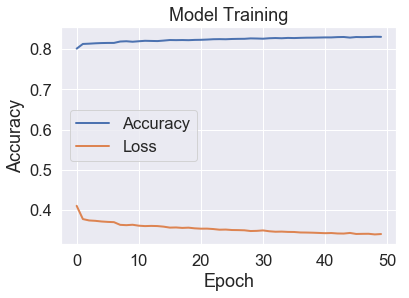Over the training epochs, the accuracy is increasing while the loss is decreasing, so that's good.

1. Since we've already one-hot encoded and scaled our test data, we can directly predict and calculate our performance. We will calculate the AUC (area-under-the-curvescore using sklearn's built-in functions. The AUC score comes from the receiver operating characteristics, which is a visualization of the false positive rate (also called the false alarm rate) on the x axis, against the true positive rate (also called the hit rate) on the y axis. The integral under this curve, the AUC score, is a popular measure of classification performance and is useful for understanding the trade-off between a high hit rate and any false alarms:
`from sklearn.metrics import roc_auc_scorepredictions = model.predict(X_test)# Please note that the targets have slightly different names in the test set than in the training dataset. We'll need to take care of this here:target_lookup = {' <=50K.': 0, ' >50K.': 1 }y_test = test['50k'].apply(    lambda x: target_lookup[x]).valuesroc_auc_score(y_test, predictions.argmax(axis=1))`

We get 0.7579310072282265 as the AUC score. An AUC score of 76% can be a good or bad score depending on the difficulty of the task. It's not bad for this dataset, but we could probably improve the performance by tweaking the model more. However, for now, we'll leave it as it is here.

1. Finally, we are going to check the feature importances. For this, we are going to use the eli5 library for black-box permutation importance. Black-box permutation importance encompasses a range of techniques that are model-agnostic, and, roughly speaking, permute features in order to establish their importance. You can read more about permuation importance in the How it works... section.

For this to work, we need a scoring function, as follows:

`from eli5.permutation_importance import get_score_importancesdef score(data, y=None, weight=None):  return model.predict(data).argmax(axis=1)base_score, score_decreases = get_score_importances(score, X_test, y_test)feature_importances = np.mean(score_decreases, axis=0).mean(axis=1)`

Now we can print the feature importances in sorted order:

`import operatorfeature_importances_annotated = {col: imp for col, imp in zip(x_cleaned_cols, feature_importances)}sorted_feature_importances_annotated = sorted(feature_importances_annotated.items(), key=operator.itemgetter(1), reverse=True)for i, (k, v) in enumerate(sorted_feature_importances_annotated):  print('{i}: {k}: {v}'.format(i=i, k=k, v=v))  if i > 9:        break`

We obtain something like the following list: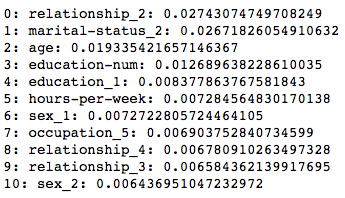Your final list might differ from the list here. The neural network training is not deterministic, although we could have tried to fix the random generator seed. Here, as we've expected, age is a significant factor; however, some categories in relationship status and marital status come up before age.

# How it works...

We went through a typical process in machine learning: we loaded a dataset, plotted and explored it, and did preprocessing with the encoding of categorical variables and normalization. We then created and trained a neural network model in Keras, and plotted the training and validation performance. Let's talk about what we did in more detail.

# Maximal information coefficient

There are many ways to calculate and plot correlation matrices, and we'll see some more possibilities in the recipes to come. Here we've calculated correlations based on the maximal information coefficient (MIC). The MIC comes from the framework of maximal information-based nonparametric exploration. This was published in Science Magazine in 2011, where it was hailed as the correlation metric of the 21st century (the article can be found at https://science.sciencemag.org/content/334/6062/1518.full).

Applied to two variables, X and Y, it heuristically searches for bins in both variables, so that the mutual information between X and Y given the bins is maximal. The coefficient ranges between 0 (no correlation) and 1 (perfect correlation). It has an advantage with respect to the Pearson correlation coefficient, firstly in that it finds correlations that are non-linear, and secondly that it works with categorical variables.

# Data generators

If you are familiar with Python generators, you won't need an explanation for what this is, but maybe a few clarifying words are in order. Using a generator gives the possibility of loading data on-demand or on-line, rather than at once. This means that you can work with datasets much larger than your available memory.

Some important terminology for generators in neural networks and Keras is as follows

• Iterations (steps_per_epoch) are the number of batches needed to complete one epoch.
• The batch size is the number of training examples in a single batch.

There are different ways to implement generators with Keras, such as the following:

• Using any Python generator
• Implementing tensorflow.keras.utils.Sequence

For the first option, we can use any generator really, but this uses a function with yield. This means we're providing the steps_per_epoch parameter for the Keras fit_generator() function.

As for the second, we write a class that inherits from tensorflow.keras.utils.Sequence, which implements the following methods:

• len(), in order for the fit_generator() function to know how much more data is to come. This corresponds to steps_per_epoch and is.
• __getitem__(), for the fit_generator to ask for the next batch.
• on_epoch_end() to do some shuffling or other things at the end of an epoch – this is optional.

For simplicity, we've taken the former approach.

We'll see later that batch data loading using generators is often a part of online learning, that is, the type of learning where we incrementally train a model on more and more data as it comes in.

# Permutation importance

The eli5 library can calculate permutation importance, which measures the increase in the prediction error when features are not present. It's also called the mean decrease accuracy (MDA). Instead of re-training the model in a leave-one-feature-out fashion, the feature can be replaced by random noise. This noise is drawn from the same distribution as the feature so as to avoid distortions. Practically, the easiest way to do this is to randomly shuffle the feature values between rows. You can find more details about permutation importance in Breiman's Random Forests (2001), at https://www.stat.berkeley.edu/%7Ebreiman/randomforest2001.pdf.

We'll cover a lot more about Keras, the underlying TensorFlow library, online learning, and generators in the recipes to come. I'd recommend you get familiar with layer types, data loaders and preprocessors, losses, metrics, and training options. All this is transferable to other frameworks such as PyTorch, where the application programming interface (API) differs; however, the essential principles are the same.

Here are links to the documentation for TensorFlow/Keras:

Both the Keras/TensorFlow combination and PyTorch provide a lot of interesting functionality that's beyond the scope of this recipe – or even this book. To name just a few, PyTorch has automatic differentiation functionality (in the form of autograd, with more info at https://pytorch.org/docs/stable/autograd.html), and TensorFlow has an estimator API, which is an abstraction similar to Keras (for more detail on this, see https://www.tensorflow.org/guide/estimator).